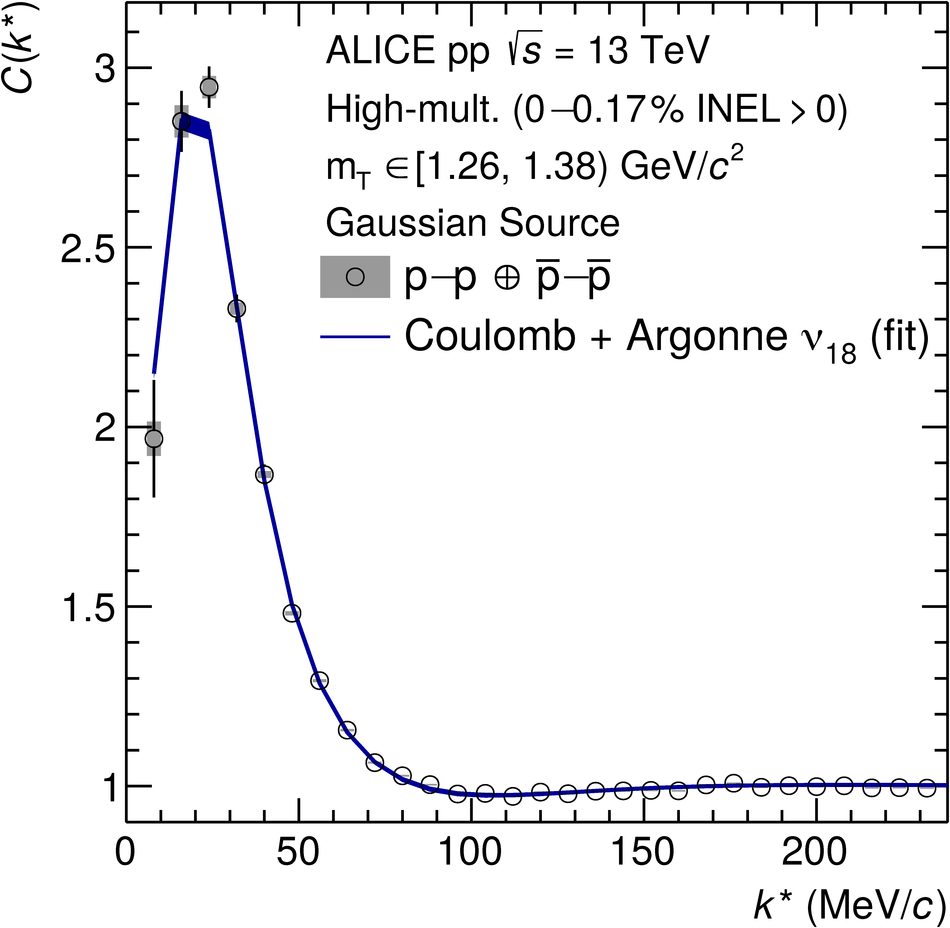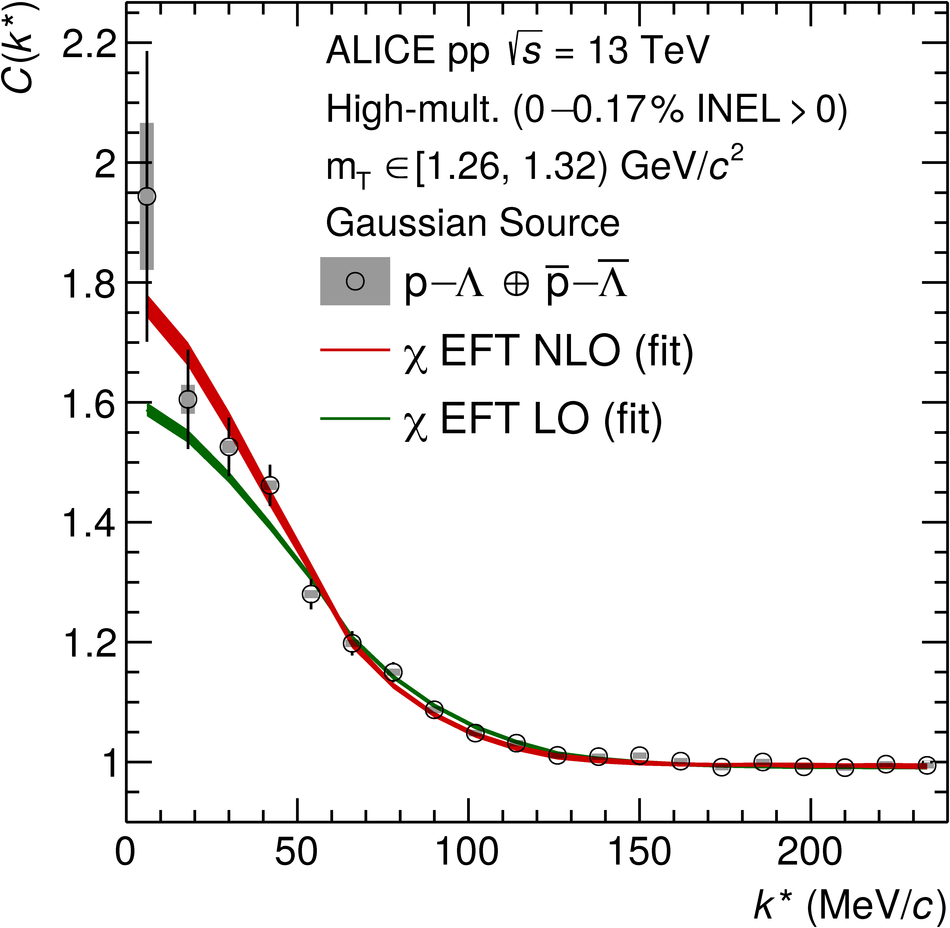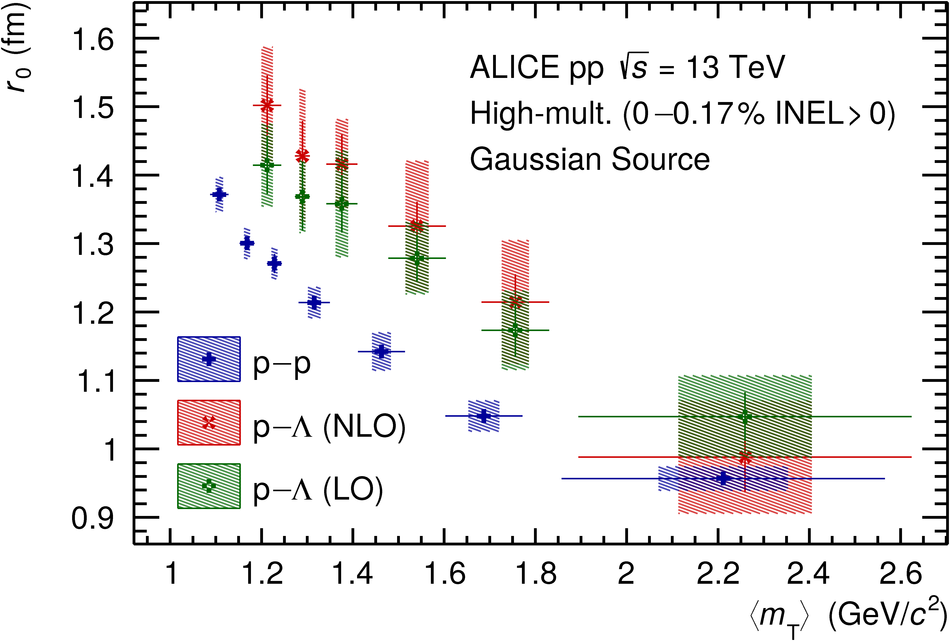# Search for a common baryon source in high-multiplicity pp collisions at the LHC

We report on the measurement of the size of the particle-emitting source from two-baryon correlations with ALICE in high-multiplicity pp collisions at $\sqrt{s}$ = 13 TeV. The source radius is studied with low relative momentum p-p, $\bar{\rm{p}}$-$\bar{\rm{p}}$, p-$\Lambda$ and $\bar{\rm{p}}$-$\bar{\Lambda}$ pairs as a function of the pair transverse mass $m_{\rm{T}}$ considering for the first time in a quantitative way the effect of strong resonance decays. After correcting for this effect, the radii extracted for pairs of different particle species agree. This indicates that protons, antiprotons, $\Lambda$, and $\bar{\Lambda}$ originate from the same source. Within the measured $m_{\rm{T}}$ range (1.1-2.2) GeV/$c^{2}$ the invariant radius of this common source varies between 0.85 and 1.3 fm. These results provide a precise reference for studies of the strong hadron-hadron interactions and for the investigation of collective properties in small colliding systems.

Submitted to: PLB
e-Print: arXiv:2004.08018 | PDF | inSPIRE
CERN-EP-2020-053

Figures

## Figure 1

 The correlation function of \pP (left) and \pL (right) as a function of \ks in one exemplary \mt interval. Statistical (bars) and systematic (boxes) uncertainties are shown separately. The filled bands depict $1\sigma$ uncertainties of the fits with $C_{\mathrm{\rm{fit}}}(\ks)$ and are obtained by using the Argonne $v_{18}$~ (blue), $\chi$EFT LO~ (green) and $\chi$EFT NLO~ (red) potentials. See text for details.## Figure 2

 Source radius r$_0$ as a function of $\langle \mt \rangle$ for the assumption of a purely Gaussian source. The blue crosses result from fitting the \pP correlation function with the strong Argonne $v_{18}$~ potential. The green squared crosses (red diagonal crosses) result from fitting the \pL correlation functions with the strong $\chi$EFT LO~ (NLO~) potential. Statistical (lines) and systematic (boxes) uncertainties are shown separately.## Figure 3

 A sketch representing the modification of $r^*_\mathrm{core}$ into $r^*$ (dash-dotted lines), due to the presence of resonances (gray disks), decaying into the particles of interest (blue disks). The coordinate system is determined by the rest frame of the two daughters and consistent with Eq.~\ref{eq:CFsourcewf}, where $k^*$ represents their momenta (solid blue lines). The blue dotted lines represent the remaining decay products, which are assumed to be single pions. In case of a primordial particle in the initial state instead of a resonance, the latter is not considered ($\vec{s}^*_\mathrm{res,i}=0$).## Figure 4

 The source functions for \pP (blue circles) and \pL (red open circles), generated by folding the exponential expansion due to the decay of the respective parent resonances with a common Gaussian core with $\rc=\SI{1.2}{fm}$ (dashed black line). Additionally shown are fits with Gaussian distributions (dotted lines) to extract the effective Gaussian source sizes.## Figure 5

 Source radius $r_{\textrm{core}}$ as a function of $\langle\mt\rangle$ for the assumption of a Gaussian source with added resonances. The blue crosses result from fitting the \pP correlation function with the strong Argonne $v_{18}$~ potential. The green squared crosses (red diagonal crosses) result from fitting the \pL correlation functions with the strong $\chi$EFT LO~ (NLO~) potential. Statistical (lines) and systematic (boxes) uncertainties are shown separately.